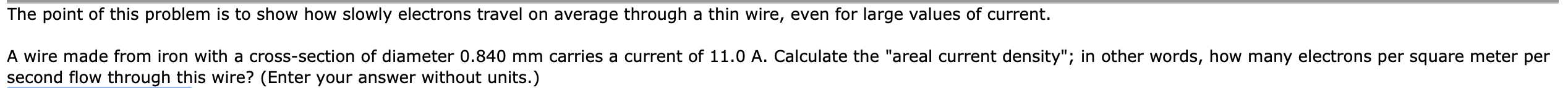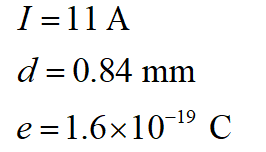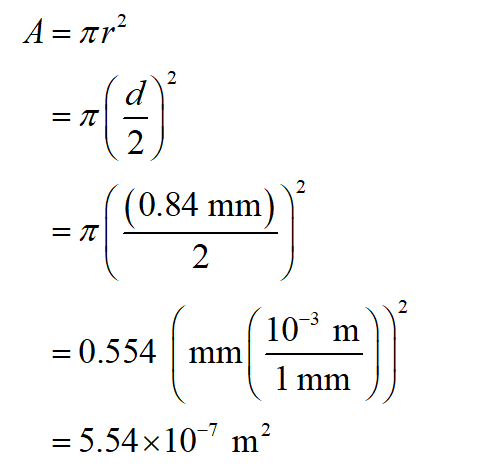# The point of this problem is to show how slowly electrons travel on average through a thin wire, even for large values of current.A wire made from iron with a cross-section of diameter 0.840 mm carries a current of 11.0 A. Calculate the "areal current density"; in other words, how many electrons per square meter persecond flow through this wire? (Enter your answer without units.)

Question
5 views

(b) Enter the larger possible value of R.help_outlineImage TranscriptioncloseThe point of this problem is to show how slowly electrons travel on average through a thin wire, even for large values of current. A wire made from iron with a cross-section of diameter 0.840 mm carries a current of 11.0 A. Calculate the "areal current density"; in other words, how many electrons per square meter per second flow through this wire? (Enter your answer without units.) fullscreen
check_circle

Step 1

Given information;Here, I is the current, d is the wire’s diameter and e is the electronic charge.

Step 2

The cross-section area (A) can be determined as,...

### Want to see the full answer?

See Solution

#### Want to see this answer and more?

Solutions are written by subject experts who are available 24/7. Questions are typically answered within 1 hour.*

See Solution
*Response times may vary by subject and question.
Tagged in